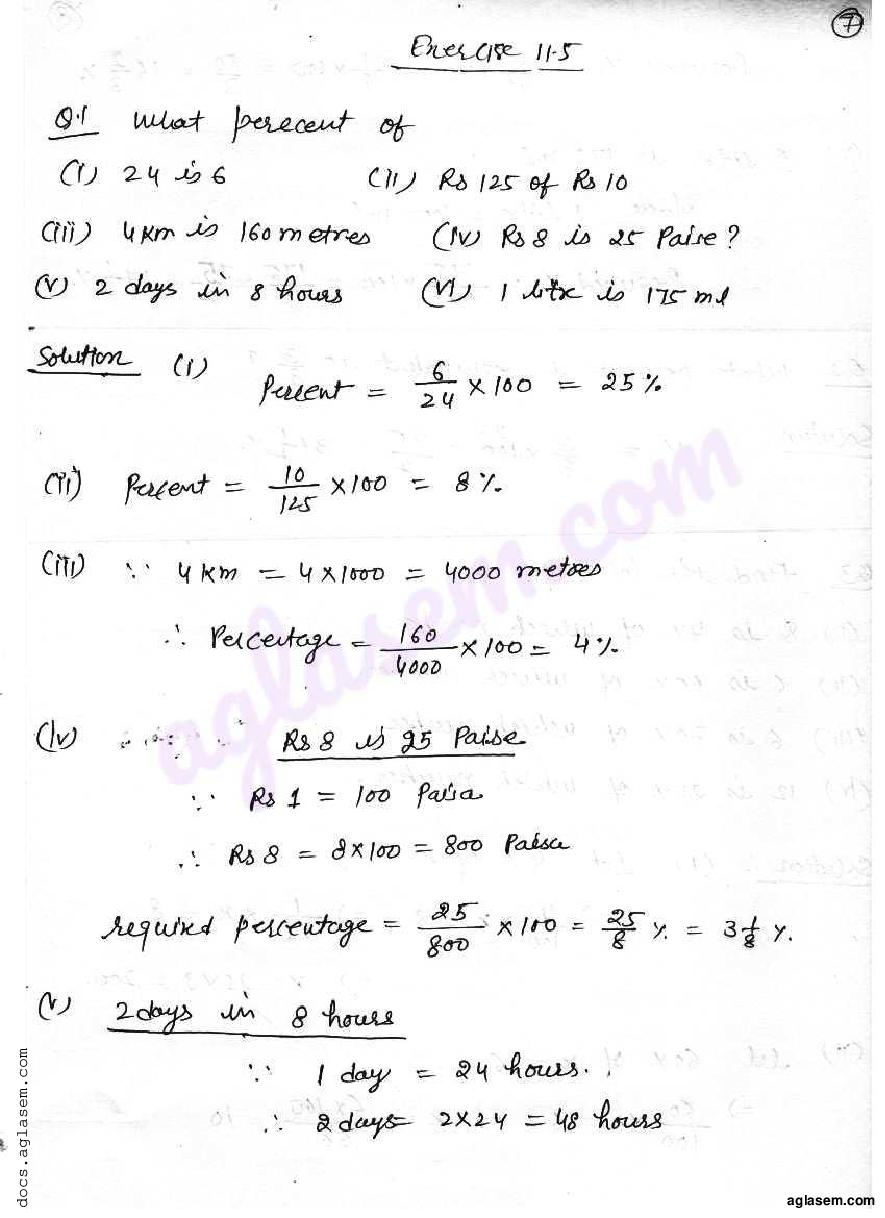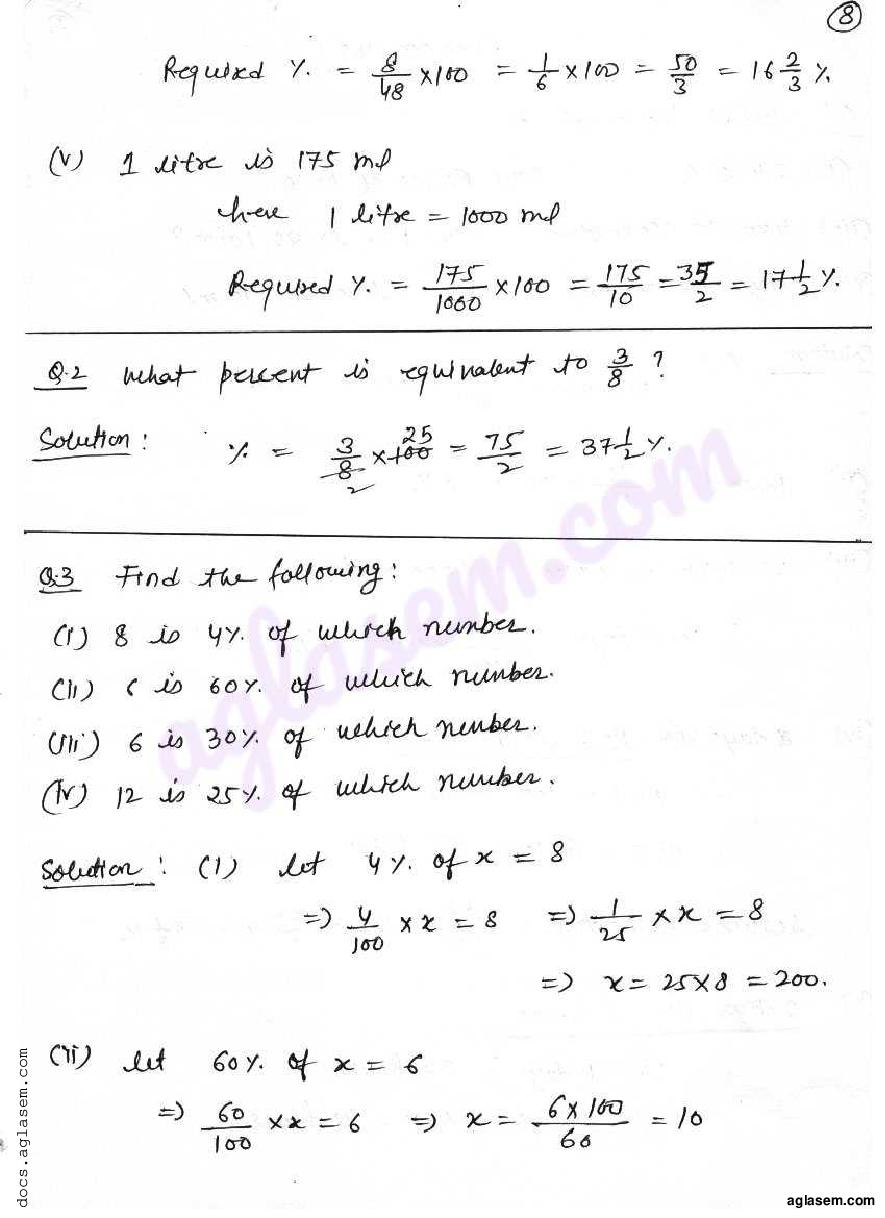# RD Sharma Solutions Class 7 Chapter 11 Percentage Exercise 11.5

Here you can get free RD Sharma Solutions for Class 7 Maths Chapter 11 Percentage Exercise 11.5. All RD Sharma Book Solutions are given here exercise wise for the chapter Percentage. RD Sharma Solutions are helpful in the preparation of several school level, graduate and undergraduate level competitive exams. Practicing questions from RD Sharma Mathematics Solutions for Class 7 Chapter 11 Percentage is proven to enhance your math skills.

 Class: Class 7th Chapter: Chapter 11 Exercise: Exercise 11.5 Name: Percentage

## RD Sharma Solutions Class 7 Chapter 11 Percentage Exercise 11.5

RD Sharma Class 7 Solutions Chapter 11 for Percentage Exercise 11.5 are given below.

RD Sharma Solutions Class 7 Chapter 11 Percentage Exercise 11.5